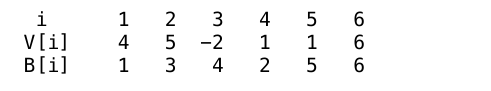# INOI 2016: Brackets

There are $k$ types of brackets each with its own opening bracket and closing bracket. We assume that the first pair is denoted by the numbers 1 and $k+1,$ the second by 2 and $k+2,$ and so on. Thus the opening brackets are denoted by $1, 2, \ldots, k,$ and the corresponding closing brackets are denoted by $k+1, k+2, \ldots, 2k,$ respectively.

Some sequences with elements from $1, 2, \ldots, 2k$ form well-bracketed sequences while others don't. A sequence is well-bracketed if we can match or pair up opening brackets of the same type in such a way that the following holds:

1. Every bracket is paired up.
2. In each matched pair, the opening bracket occurs before the closing bracket.
3. For a matched pair, any other matched pair lies either completely between them or outside them.

In this problem, you are given a sequence of brackets of length $N$: $B, \ldots, B[N]$, where each $B[i]$ is one of the brackets. You are also given an array of Values: $V,\ldots, V[N]$.

Among all the subsequences in the Values array, such that the corresponding bracket subsequence in the B Array is a well-bracketed sequence, you need to find the maximum sum.

Task: Solve the above problem for this input.

Input Format

One line, which contains $(2\times N + 2)$ space separate integers. The first integer denotes $N.$ The next integer is $k.$ The next $N$ integers are $V,..., V[N].$ The last $N$ integers are $B,..., B[N].$

Constraints

• $1 \leq k \leq 7$
• $-10^6 \leq V[i] \leq 10^6$, for all $i$
• $1 \leq B[i] \leq 2k$, for all $i$

Illustrated Examples

• For the examples discussed here, let us assume that $k = 2$. The sequence 1, 1, 3 is not well-bracketed as one of the two 1's cannot be paired. The sequence 3, 1, 3, 1 is not well-bracketed as there is no way to match the second 1 to a closing bracket occurring after it. The sequence 1, 2, 3, 4 is not well-bracketed as the matched pair 2, 4 is neither completely between the matched pair 1, 3 nor completely outside of it. That is, the matched pairs cannot overlap. The sequence 1, 2, 4, 3, 1, 3 is well-bracketed. We match the first 1 with the first 3, the 2 with the 4, and the second 1 with the second 3, satisfying all the 3 conditions. If you rewrite these sequences using [, {, ], } instead of 1, 2, 3, 4 respectively, this will be quite clear.

• Suppose $N = 6, k = 3,$ and the values of $V$ and $B$ are as follows:Then, the brackets in positions 1, 3 form a well-bracketed sequence (1, 4) and the sum of the values in these positions is 2 (4 + (-2) =2). The brackets in positions 1, 3, 4, 5 form a well-bracketed sequence (1, 4, 2, 5) and the sum of the values in these positions is 4. Finally, the brackets in positions 2, 4, 5, 6 form a well-bracketed sequence (3, 2, 5, 6) and the sum of the values in these positions is 13. The sum of the values in positions 1, 2, 5, 6 is 16 but the brackets in these positions (1, 3, 5, 6) do not form a well-bracketed sequence. You can check the best sum from positions whose brackets form a well-bracketed sequence is 13.

×